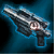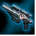# [Ranged Attack]Conditions

## Related effects, buffs and debuffs

• [0s] [not displayed]Effect #1

 Slot: Debuff Duration: 0s Tick rate: does not tick # occurrences: 0
• On Apply

Only when the following conditions are met:

• <
AND
AND
>

Perform the following actions:

• Weapon Damage
- Is Special Ability = (bool) false
- Ignore Dual Wield Modifier = (bool) true
- Unknown (609) = (bool) false
- Flurry Blows Min = (int) 1
- Flurry Blows Max = (int) 3
- Level Cap = (int) 0
- Threat Percent = (float) 0
- Standard Health Percent Max = (float) 0
- Standard Health Percent Min = (float) 0
- Amount Modifier Fixed Max = (float) 0
- Amount Modifier Fixed Min = (float) 0
- Amount Modifier Percent = (float) 0
- Coefficient = (float) 0
- Health Steal Percentage = (float) 0
- Slots = Int(2)
• Play appearance epp . flurry . npc . ranged
• On Apply

Only when the following conditions are met:

• If TARGET has an effect with the tag tag.​abl.​trooper.​ap_clip

Perform the following actions:

• Weapon Damage
- Is Special Ability = (bool) false
- Ignore Dual Wield Modifier = (bool) true
- Unknown (609) = (bool) false
- Flurry Blows Min = (int) 1
- Flurry Blows Max = (int) 3
- Level Cap = (int) 0
- Threat Percent = (float) 0
- Standard Health Percent Max = (float) 0
- Standard Health Percent Min = (float) 0
- Amount Modifier Fixed Max = (float) 0
- Amount Modifier Fixed Min = (float) 0
- Amount Modifier Percent = (float) 0
- Coefficient = (float) 0
- Health Steal Percentage = (float) 0
- Slots = Int(2)
• Play appearance epp . flurry . npc . ranged_ap
• On Apply

Only when the following conditions are met:

• If TARGET has an effect with the tag tag.​abl.​trooper.​ion_clip

Perform the following actions:

• Weapon Damage
- Is Special Ability = (bool) false
- Ignore Dual Wield Modifier = (bool) true
- Unknown (609) = (bool) false
- Flurry Blows Min = (int) 1
- Flurry Blows Max = (int) 3
- Level Cap = (int) 0
- Threat Percent = (float) 0
- Standard Health Percent Max = (float) 0
- Standard Health Percent Min = (float) 0
- Amount Modifier Fixed Max = (float) 0
- Amount Modifier Fixed Min = (float) 0
- Amount Modifier Percent = (float) 0
- Coefficient = (float) 0
- Health Steal Percentage = (float) 0
- Slots = Int(2)
• Play appearance epp . flurry . npc . ranged_ion
• On Apply

Only when the following conditions are met:

• If TARGET has an effect with the tag tag.​abl.​trooper.​plasma_clip

Perform the following actions:

• Weapon Damage
- Is Special Ability = (bool) false
- Ignore Dual Wield Modifier = (bool) true
- Unknown (609) = (bool) false
- Flurry Blows Min = (int) 1
- Flurry Blows Max = (int) 3
- Level Cap = (int) 0
- Threat Percent = (float) 0
- Standard Health Percent Max = (float) 0
- Standard Health Percent Min = (float) 0
- Amount Modifier Fixed Max = (float) 0
- Amount Modifier Fixed Min = (float) 0
- Amount Modifier Percent = (float) 0
- Coefficient = (float) 0
- Health Steal Percentage = (float) 0
- Slots = Int(2)
• Play appearance epp . flurry . npc . ranged_plasma